Continuous Random Variables

# 27 The Uniform Distribution

The uniform distribution is a continuous probability distribution and is concerned with events that are equally likely to occur. When working out problems that have a uniform distribution, be careful to note if the data is inclusive or exclusive of endpoints.

The mathematical statement of the uniform distribution is

f(x) =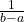for axb

where a = the lowest value of x and b = the highest value of x.

Formulas for the theoretical mean and standard deviation are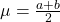and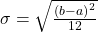Try It

The data that follow are the number of passengers on 35 different charter fishing boats. The sample mean = 7.9 and the sample standard deviation = 4.33. The data follow a uniform distribution where all values between and including zero and 14 are equally likely. State the values of a and b. Write the distribution in proper notation, and calculate the theoretical mean and standard deviation.

 1 12 4 10 4 14 11 7 11 4 13 2 4 6 3 10 0 12 6 9 10 5 13 4 10 14 12 11 6 10 11 0 11 13 2

a is zero; b is 14; X ~ U (0, 14); μ = 7 passengers; σ = 4.04 passengers

The amount of time, in minutes, that a person must wait for a bus is uniformly distributed between zero and 15 minutes, inclusive.

a. What is the probability that a person waits fewer than 12.5 minutes?

a. Let X = the number of minutes a person must wait for a bus. a = 0 and b = 15. X ~ U(0, 15). Write the probability density function. f (x) =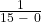=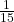for 0 ≤ x ≤ 15.

Find P (x < 12.5). Draw a graph.The probability a person waits less than 12.5 minutes is 0.8333.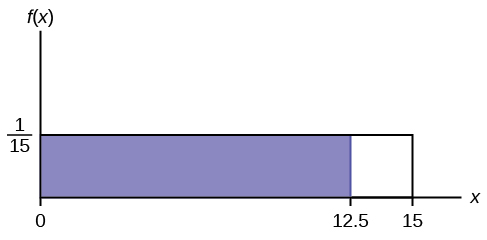b. On the average, how long must a person wait? Find the mean, μ, and the standard deviation, σ.

b. μ =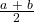=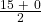= 7.5. On the average, a person must wait 7.5 minutes.

σ =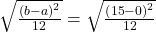= 4.3. The Standard deviation is 4.3 minutes.

c. Ninety percent of the time, the time a person must wait falls below what value?

NOTE

This asks for the 90th percentile.

c. Find the 90th percentile. Draw a graph. Let k = the 90th percentile.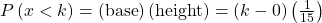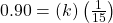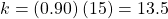The 90th percentile is 13.5 minutes. Ninety percent of the time, a person must wait at most 13.5 minutes.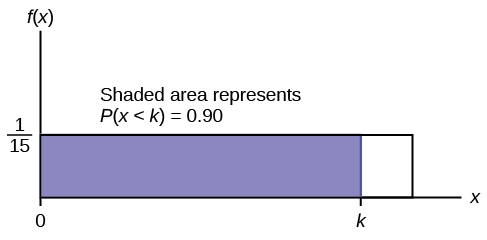Try It

The total duration of baseball games in the major league in the 2011 season is uniformly distributed between 447 hours and 521 hours inclusive.

1. Find a and b and describe what they represent.
2. Write the distribution.
3. Find the mean and the standard deviation.
4. What is the probability that the duration of games for a team for the 2011 season is between 480 and 500 hours?
1. a is 447, and b is 521. a is the minimum duration of games for a team for the 2011 season, and b is the maximum duration of games for a team for the 2011 season.
2. X ~ U (447, 521).
3. μ = 484, and σ = 21.36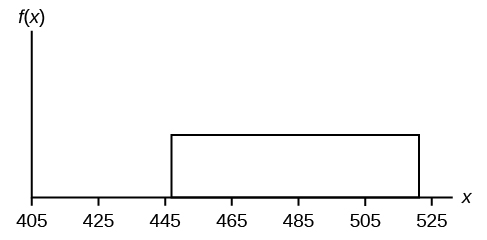4. P(480 < x < 500) = 0.2703

### Chapter Review

If X has a uniform distribution where a < x < b or axb, then X takes on values between a and b (may include a and b). All values x are equally likely. We write XU(a, b). The mean of X is. The standard deviation of X is. The probability density function of X is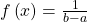for axb. The cumulative distribution function of X is P(Xx) =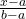. X is continuous.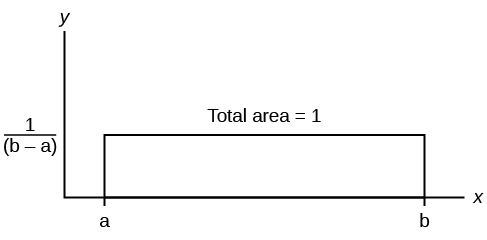The probability P(c < X < d) may be found by computing the area under f(x), between c and d. Since the corresponding area is a rectangle, the area may be found simply by multiplying the width and the height.

### Formula Review

X = a real number between a and b (in some instances, X can take on the values a and b). a = smallest X; b = largest X

X ~ U (a, b)

The mean isThe standard deviation is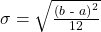Probability density function:for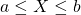Area to the Left of x:P(X < x) = (xa)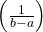Area to the Right of x:P(X > x) = (bx)Area Between c and d:P(c < x < d) = (base)(height) = (dc)• pdf:for a ≤ x ≤ b
• cdf: P(Xx) =• mean µ =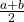• standard deviation σ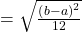• P(c < X < d) = (dc)### References

McDougall, John A. The McDougall Program for Maximum Weight Loss. Plume, 1995.

Use the following information to answer the next ten questions. The data that follow are the square footage (in 1,000 feet squared) of 28 homes.

 1.5 2.4 3.6 2.6 1.6 2.4 2 3.5 2.5 1.8 2.4 2.5 3.5 4 2.6 1.6 2.2 1.8 3.8 2.5 1.5 2.8 1.8 4.5 1.9 1.9 3.1 1.6

The sample mean = 2.50 and the sample standard deviation = 0.8302.

The distribution can be written as X ~ U(1.5, 4.5).

What type of distribution is this?

In this distribution, outcomes are equally likely. What does this mean?

It means that the value of x is just as likely to be any number between 1.5 and 4.5.

What is the height of f(x) for the continuous probability distribution?

What are the constraints for the values of x?

1.5 ≤ x ≤ 4.5

Graph P(2 < x < 3).

What is P(2 < x < 3)?

0.3333

What is P(x < 3.5x < 4)?

What is P(x = 1.5)?

zero

Find the probability that a randomly selected home has more than 3,000 square feet given that you already know the house has more than 2,000 square feet.

0.6

Use the following information to answer the next eight exercises. A distribution is given as X ~ U(0, 12).

What is a? What does it represent?

What is b? What does it represent?

b is 12, and it represents the highest value of x.

What is the probability density function?

What is the theoretical mean?

six

What is the theoretical standard deviation?

Draw the graph of the distribution for P(x > 9).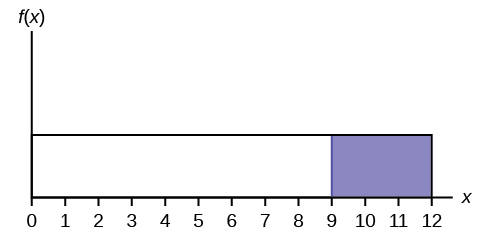Find P(x > 9).

Use the following information to answer the next eleven exercises. The age of cars in the staff parking lot of a suburban college is uniformly distributed from six months (0.5 years) to 9.5 years.

What is being measured here?

In words, define the random variable X.

X = The age (in years) of cars in the staff parking lot

Are the data discrete or continuous?

The interval of values for x is ______.

0.5 to 9.5

The distribution for X is ______.

Write the probability density function.

f(x) =where x is between 0.5 and 9.5, inclusive.

Graph the probability distribution.

1. Sketch the graph of the probability distribution.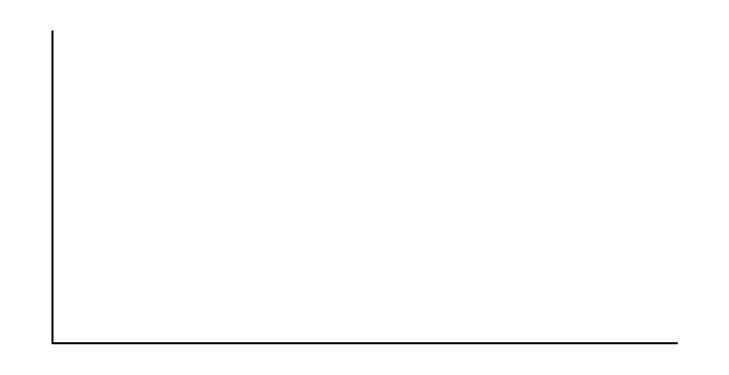2. Identify the following values:
1. Lowest value for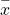: _______
2. Highest value for: _______
3. Height of the rectangle: _______
4. Label for x-axis (words): _______
5. Label for y-axis (words): _______

Find the average age of the cars in the lot.

μ = 5

Find the probability that a randomly chosen car in the lot was less than four years old.

1. Sketch the graph, and shade the area of interest.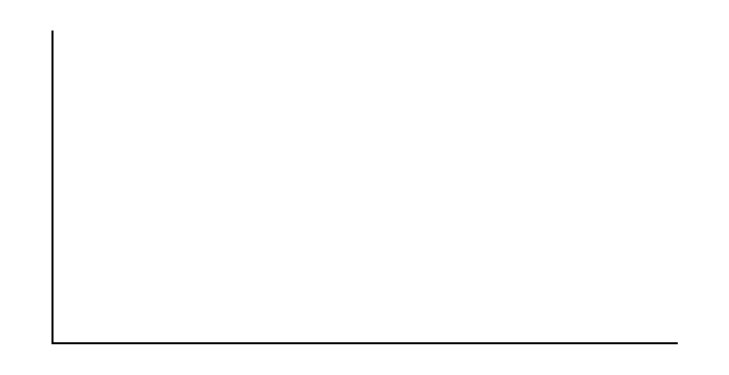2. Find the probability. P(x < 4) = _______

Considering only the cars less than 7.5 years old, find the probability that a randomly chosen car in the lot was less than four years old.

1. Sketch the graph, shade the area of interest.2. Find the probability. P(x < 4x < 7.5) = _______
1. Check student’s solution.
2.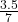What has changed in the previous two problems that made the solutions different?

Find the third quartile of ages of cars in the lot. This means you will have to find the value such that, or 75%, of the cars are at most (less than or equal to) that age.

1. Sketch the graph, and shade the area of interest.2. Find the value k such that P(x < k) = 0.75.
3. The third quartile is _______
1. Check student’s solution.
2. k = 7.25
3. 7.25

### Homework

For each probability and percentile problem, draw the picture.

Births are approximately uniformly distributed between the 52 weeks of the year. They can be said to follow a uniform distribution from one to 53 (spread of 52 weeks).

1. Graph the probability distribution.
2. f(x) = _________
3. μ = _________
4. σ = _________
5. Find the probability that a person is born at the exact moment week 19 starts. That is, find P(x = 19) = _________
6. P(2 < x < 31) = _________
7. Find the probability that a person is born after week 40.
8. P(12 < xx < 28) = _________

A random number generator picks a number from one to nine in a uniform manner.

1. Graph the probability distribution.
2. f(x) = _________
3. μ = _________
4. σ = _________
5. P(3.5 < x < 7.25) = _________
6. P(x > 5.67)
7. P(x > 5x > 3) = _________
1. Check student’s solution.
2.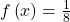where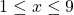3. five
4. 2.3
5.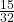6.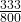7.According to a study by Dr. John McDougall of his live-in weight loss program at St. Helena Hospital, the people who follow his program lose between six and 15 pounds a month until they approach trim body weight. Let’s suppose that the weight loss is uniformly distributed. We are interested in the weight loss of a randomly selected individual following the program for one month.

1. Define the random variable. X = _________
2. Graph the probability distribution.
3. f(x) = _________
4. μ = _________
5. σ = _________
6. Find the probability that the individual lost more than ten pounds in a month.
7. Suppose it is known that the individual lost more than ten pounds in a month. Find the probability that he lost less than 12 pounds in the month.
8. P(7 < x < 13x > 9) = __________. State this in a probability question, similarly to parts g and h, draw the picture, and find the probability.

A subway train on the Red Line arrives every eight minutes during rush hour. We are interested in the length of time a commuter must wait for a train to arrive. The time follows a uniform distribution.

1. Define the random variable. X = _______
2. Graph the probability distribution.
3. f(x) = _______
4. μ = _______
5. σ = _______
6. Find the probability that the commuter waits less than one minute.
7. Find the probability that the commuter waits between three and four minutes.
1. X represents the length of time a commuter must wait for a train to arrive on the Red Line.
2. Graph the probability distribution.
3.where 0 ≤ x ≤ 8
4. four
5. 2.31
6.7.The age of a first grader on September 1 at Garden Elementary School is uniformly distributed from 5.8 to 6.8 years. We randomly select one first grader from the class.

1. Define the random variable. X = _________
2. Graph the probability distribution.
3. f(x) = _________
4. μ = _________
5. σ = _________
6. Find the probability that she is over 6.5 years old.
7. Find the probability that she is between four and six years old.

Use the following information to answer the next three exercises. The Sky Train from the terminal to the rental–car and long–term parking center is supposed to arrive every eight minutes. The waiting times for the train are known to follow a uniform distribution.

What is the average waiting time (in minutes)?

1. zero
2. two
3. three
4. four

d

The probability of waiting more than seven minutes given a person has waited more than four minutes is?

1. 0.125
2. 0.25
3. 0.5
4. 0.75

b

The time (in minutes) until the next bus departs a major bus depot follows a distribution with f(x) =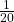where x goes from 25 to 45 minutes.

1. Define the random variable. X = ________
2. Graph the probability distribution.
3. The distribution is ______________ (name of distribution). It is _____________ (discrete or continuous).
4. μ = ________
5. σ = ________
6. Find the probability that the time is at most 30 minutes. Sketch and label a graph of the distribution. Shade the area of interest. Write the answer in a probability statement.
7. Find the probability that the time is between 30 and 40 minutes. Sketch and label a graph of the distribution. Shade the area of interest. Write the answer in a probability statement.
8. P(25 < x < 55) = _________. State this in a probability statement, similarly to parts g and h, draw the picture, and find the probability.

Suppose that the value of a stock varies each day from ?16 to ?25 with a uniform distribution.

1. Find the probability that the value of the stock is more than ?19.
2. Find the probability that the value of the stock is between ?19 and ?22.
3. Given that the stock is greater than ?18, find the probability that the stock is more than ?21.
1. The probability density function of X is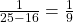.
P(X > 19) = (25 – 19)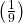==.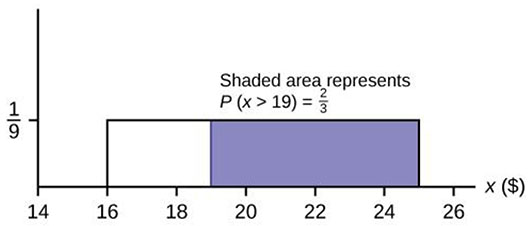2. P(19 < X < 22) = (22 – 19)==.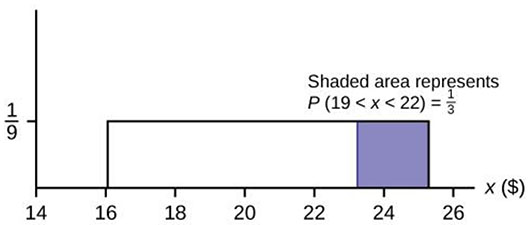3. This is a conditional probability question. P(x > 21x > 18). You can do this two ways:
• Draw the graph where a is now 18 and b is still 25. The height is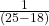=So, P(x > 21x > 18) = (25 – 21)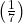= 4/7.
• Use the formula: P(x > 21x > 18) =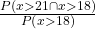=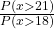=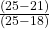=.

A fireworks show is designed so that the time between fireworks is between one and five seconds, and follows a uniform distribution.

1. Find the average time between fireworks.
2. Find probability that the time between fireworks is greater than four seconds.

The number of miles driven by a truck driver falls between 300 and 700, and follows a uniform distribution.

1. Find the probability that the truck driver goes more than 650 miles in a day.
2. Find the probability that the truck drivers goes between 400 and 650 miles in a day.
1. P(X > 650) =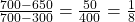= 0.125.
2. P(400 < X < 650) =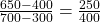= 0.625

### Key Terms

Conditional Probability
the likelihood that an event will occur given that another event has already occurred.